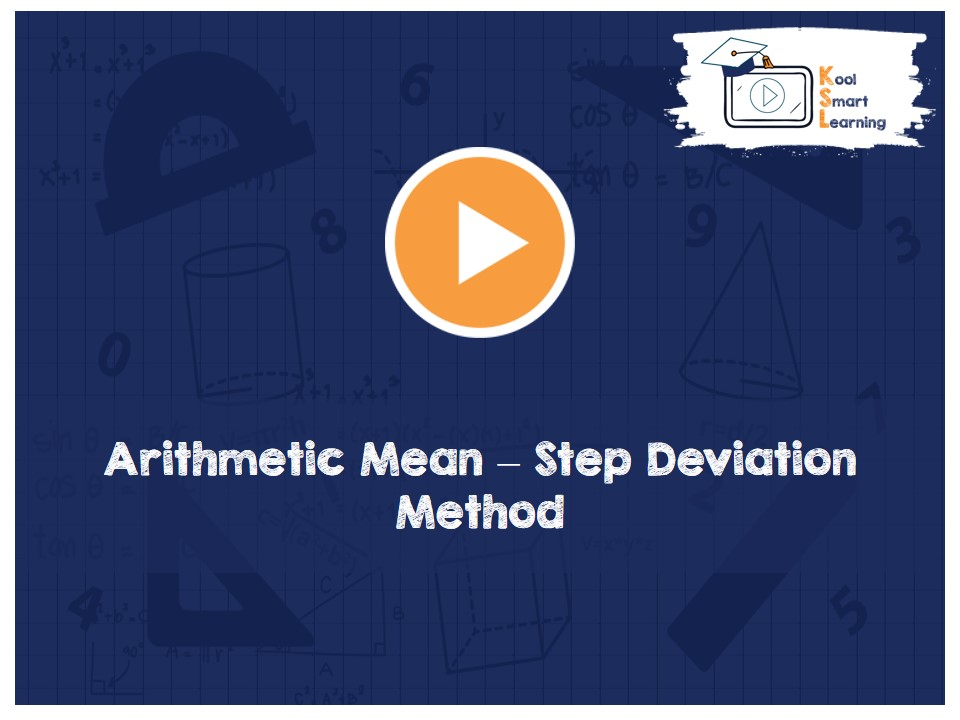At KoolSmartLearning, we intend to harness the power of online education to make learning easy.## Arithmetic Mean – Step Deviation Method

/  Arithmetic Mean – Step Deviation Method## Arithmetic Mean – Step Deviation Method

This video talks about calculating Arithmetic Mean of grouped data using Step-Deviation Method. This method is in a way extension of assumed mean method. The assumed mean is divided with a number and then the calculation of the arithmetic mean is done using that. It explains how assuming different values of mean & the number by which the assumed mean is divided will not change the result.

More Related Videos